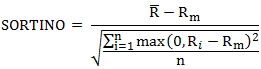# SQL Server Sortino ratio

SORTINO

Updated: 04 April 2015

Use the aggregate function SORTINO to calculate the Sortino ratio based upon return data. The Sortino ratio is calculated as the mean difference of the returns (R) and the minimum acceptable return (Rm) divided by the downside deviation.Where

 R¯ = the average of the returns Rm = the minimum acceptable return Ri = the ith return n = When @Full = 'TRUE' then the number of rows passed into the function; else the number of rows where max(0, Ri – Rm) < 0.

Syntax
SELECT [wct].[SORTINO](
<@R, float,>
,<@Mar, float,>
,<@Full, bit,>)
Arguments
@R
the return for the period; the percentage return in floating point format (i.e. 10% = 0.10). @R is an expression of type float or of a type that can be implicitly converted to float.
@Mar
the minimum acceptable return. @Mar is an expression of type float or of a type that can be implicitly converted to float.
@Full
a bit value which defines how to calculate the downside deviation. When @Full = 'TRUE' the downside deviation is calculated with n equal to the number of rows passed into the function. When @Full = 'FALSE' n is equal to the number of rows where max(0,R – Rm) < 0.
Return Type
float
Remarks
·         If there are no negative returns, then SORTINO is NULL.
·         @Mar must be the same for all rows in the GROUP BY.
·         @Full must be the same for all rows in the GROUP BY.
·         @Full defaults to'FALSE'.
·         Available in XLeratorDB / financial 2008 only
Examples
In this example, we take 12 monthly returns. Our minimum acceptable return is 10% per year, which we turn into a monthly return using the formula 1.101/12-1. The downside deviation is calculated with n = 12.
DECLARE @MAR as float = POWER(1.1e+00,1E+00/12e+00)-1

SELECT
wct.SORTINO(
R
,@MAR
,'TRUE'
) as Sortino
FROM (VALUES
(1,0.0089)
,(2,0.0012)
,(3,-0.002)
,(4,0.01)
,(5,-0.0002)
,(6,0.02)
,(7,0.03)
,(8,0.01)
,(9,-0.003)
,(10,0.01)
,(11,0.0102)
,(12,-0.01)
)n(mth,r)

This produces the following result.

Using the same data as above, we change @Full =
'FALSE' and the downside deviation is calculated with n = 5.
DECLARE @MAR as float = POWER(1.1e+00,1E+00/12e+00)-1

SELECT
wct.SORTINO(
R
,@MAR
,'FALSE'
) as Sortino
FROM (VALUES
(1,0.0089)
,(2,0.0012)
,(3,-0.002)
,(4,0.01)
,(5,-0.0002)
,(6,0.02)
,(7,0.03)
,(8,0.01)
,(9,-0.003)
,(10,0.01)
,(11,0.0102)
,(12,-0.01)
)n(mth,r)

This produces the following result.Copyright 2008-2021 Westclintech LLC         Privacy Policy        Terms of Service